# 1. 问题描述

“红线”是马塞诸塞州了解剑桥和波士顿的地铁线路，在上下班高峰期，红线列车每 7 ~ 8 分钟运行一趟。从 Kendall 广场乘坐红线列车，在到达车站时，可以根据站台上的乘客人数估计下一班车到达的时间：如果站台上只有几个人，说明可能刚过去了一趟列车，下一班要等 7 分钟左右；如果站台上有较多的乘客等待，则估计下一班车班上就回到来；如果站台上的人很多，则列车可能没有正常运行，最好去打出租。通过贝叶斯估计，根据站台上的等待人数预测等待时间，并决定什么时候最好改乘出租车。

# 2. 模型

假设乘客抵达车站是一个泊松过程，即乘客等概率地可能在任何时间点到达车站。记乘客进站的速率，即每分钟进入车站的乘客数量为 $\lambda$，假设它是一个未知的常数。

高峰期间，列车从始发站每隔 7 ~ 8 分钟发出，到达 Kendall 广场站时，列车的间隔在 3 ~ 12 分钟之间变化。收集每个工作日下午 4 点到 6 点列车发车间隔的数据 OBSERVED_GAP_TIMES 如下（单位为秒）：

OBSERVED_GAP_TIMES = [
428.0, 705.0, 407.0, 465.0, 433.0, 425.0, 204.0, 506.0, 143.0, 351.0,
450.0, 598.0, 464.0, 749.0, 341.0, 586.0, 754.0, 256.0, 378.0, 435.0,
176.0, 405.0, 360.0, 519.0, 648.0, 374.0, 483.0, 537.0, 578.0, 534.0,
577.0, 619.0, 538.0, 331.0, 186.0, 629.0, 193.0, 360.0, 660.0, 484.0,
512.0, 315.0, 457.0, 404.0, 740.0, 388.0, 357.0, 485.0, 567.0, 160.0,
428.0, 387.0, 901.0, 187.0, 622.0, 616.0, 585.0, 474.0, 442.0, 499.0,
437.0, 620.0, 351.0, 286.0, 373.0, 232.0, 393.0, 745.0, 636.0, 758.0,
]

定义 get_sample_pmf() 根据指定数据计算 PMF：

def get_sample_pmf(gap_times=OBSERVED_GAP_TIMES, name=''):
xs = make_range(low=10)
pdf = EstimatedPdf(gap_times)
pmf = pdf.make_pmf(xs, name=name)
return pmf

def make_range(low=10, high=1200, skip=30):
return range(low, high + skip, skip)

get_sample_pmf() 中的 EstimatedPdf 使用高斯核给出核密度估计，然后转换为离散的 PMF 的形式。make_range() 是一个生成时间区间的工具方法，默认生成从 10 秒（low）到 1200 秒（high）、间隔为 30 秒（skip）的区间。

使用 get_sample_pmf() 可以得到工作日下午 4 点到 6 点的发车间隔 PMF，如果你也在每个工作日下午 4 点到 6 点间在站台记录列车间隔，你会得到同样的结果。但如果你随机地到达车站，则会得到不同的分布。为了说明这一点，假设列车会按 5 分钟或 10 分钟（等概率）的间隔发车，此时列车的平均间隔时间是 $0.5 \times 5 + 0.5 \times 10 = 7.5$ 分钟。而乘客会在任意时刻等可能地到达车站，这意味着有 $\frac{5}{5 + 10} = \frac{1}{3}$ 的乘客会等到 5 分钟发车间隔的列车，这些乘客所观察到的列车间隔为 5 分钟；而有 $\frac{10}{5 + 10} = \frac{2}{3}$ 的乘客会等到 10 分钟发车间隔的列车，这些乘客所观察到的列车间隔为 10 分钟。对于乘客来说，他们所观察到的平均列车间隔为 $\frac{1}{3} \times 5 + \frac{2}{3} \times 10 \approx 8.33$ 分钟，大于实际列车发车间隔。这称为观察者偏差。

乘客所观察到的列车间隔，是列车的实际发车间隔按照其对应概率进行采样的结果，定义 bias_pmf 计算乘客观察到的偏差的列车发车间隔 PMF：

def bias_pmf(pmf, name=''):
pmf_new = pmf.copy(name=name)
for x, p in pmf.iter_items():
pmf_new.mult(x, x)
pmf_new.normalize()
return pmf_new

bias_pmf() 中，对于原始 pmf 中的每个发车间隔和概率，更新概率为概率乘以发车间隔。对于乘客来说，发车间越长的列车越容易被观测到。

使用如下代码计算并比较实际的列车间隔（pmf_z）和乘客观察到的列车间隔（pmf_zb）：

pmf_z = get_sample_pmf(name='z')
pmf_zb = bias_pmf(pmf_z, name='zb')
pmf_z.plot_with([pmf_zb])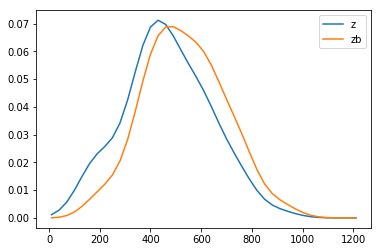# 3. 等待时间

记乘客到站后，上一趟列车已经开走的时间为 $x$，乘客等待下一趟列车的时间为 $y$，乘客观察到的的列车间隔为 $zb$，则有：

\begin{equation}
zb = x + y
\end{equation}

继续前面的例子，乘客有 $\frac{1}{3}$ 的概率观察到 5 分钟间隔的列车，$\frac{2}{3}$ 的概率观察到 10 分钟间隔的列车，即 $zb$ 为 5 的概率是 $\frac{1}{3}$，为 10 的概率是 $\frac{2}{3}$。由于乘客在任意时刻等可能地到达车站，则对于 5 分钟间隔的列车，乘客在 5 分钟内随机到达，其等待时间 $y$ 均匀分布在 $[0, 5]$ 的区间内；同理，对于 10 分钟间隔的列车，$y$ 均匀分布在 $[0, 10]$ 的区间内，$y$ 在整体上的分布是以各个间隔的概率进行加权的若干个均匀分布组合而成的。由此，可以根据给定的 $zb$ 的分布，计算得到 $y$ 的分布。

定义 pmf_of_wait_time() 计算等待时间 $y$ 的 PMF：

def pmf_of_wait_time(pmf_zb):
pmf_meta = Pmf()
for gap, prob in pmf_zb.iter_items():
uniform = make_uniform_pmf(0, gap)
pmf_meta.set(uniform, prob)
pmf_y = make_mixture(pmf_meta)
return pmf_y

def make_uniform_pmf(low, high):
pmf = Pmf()
for x in make_range(low, high):
pmf.set(x, 1)
pmf.normalize()
return pmf

pmf_of_wait_time()pmf_zb 中的每一个条目通过 make_uniform_pmf() 创建了一个均匀分布，将这个均匀分布及其概率加入 pmf_meta，通过 make_mixture 构造混合分布。

根据前面的 pmf_zb 计算 $y$ 的分布：

pmf_y = pmf_of_wait_time(pmf_zb)
pmf_y.plot()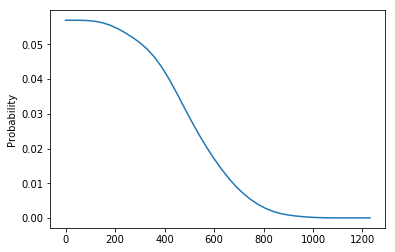定义 WaitTimeCalculator 统一计算 $z$、$zb$ 和 $y$ 的分布：

class WaitTimeCalculator(object):
def __init__(self, pmf_z):
self.pmf_z = pmf_z
self.pmf_zb = bias_pmf(pmf_z)
self.pmf_y = pmf_of_wait_time(self.pmf_zb)
self.pmf_x = self.pmf_y

def plot_cdf(self):
cdf_z = self.pmf_z.make_cdf(name='z')
cdf_zb = self.pmf_zb.make_cdf(name='zb')
cdf_y = self.pmf_y.make_cdf(name='y')
cdf_z.plot_with([cdf_zb, cdf_y])

计算 $z$、$zb$ 和 $y$ 的分布，并绘制 CDF：

wtc = WaitTimeCalculator(pmf_z)
print("z mean: {:.1f} min\nzb mean: {:.1f} min\ny mean: {:.1f} min"
.format(wtc.pmf_z.mean()/60, wtc.pmf_zb.mean()/60, wtc.pmf_y.mean()/60))
wtc.plot_cdf()

z mean: 7.8 min
zb mean: 8.9 min
y mean: 4.6 min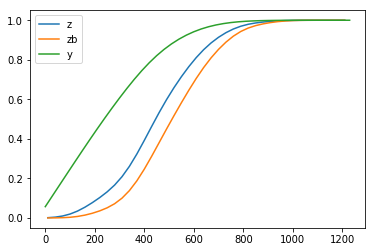# 4. 预测等待时间

假设我们通过在车站观察人流，得知乘客进站速率 $\lambda$ 为 2 人/分钟，即每分钟有 2 名乘客进站。现在车站有 15 名乘客正在候车，则预计的等待时间是多久呢？

在上一步中，我们通过收集数据得到了列车实际间隔的分布 pmf_z，并得到了乘客所观察到的列车间隔的分布 pmf_zb 和上一班车已经开走的时间 $x$ 的分布 pmf_x（先验分布）。现在，我们观察到车站有 15 名乘客，就可以计算得到 $x$ 的后验分布，并通过 $y = zb – x$ 得到 $y$ 的分布，即等待时间的分布。

定义 Elapsed 表示上一班车已经开走的时间（$x$）：

class Elapsed(Suite):
def likelihood(self, data, hypo):
x = hypo
lam, k = data
like = eval_poisson_pmf(lam * x, k)
return like

likelihood() 的参数 data 为乘客进站速率（lam）和当前车站候车人数（k）的元组，hypo 为上一班车已经开走的时间 $x$ 的假设。

定义 ElapsedTimeEstimator 估计上一班车开走的时间：

class ElapsedTimeEstimator(object):
def __init__(self, wtc, lam=2.0/60, num_passengers=15):
self.prior_x = Elapsed(wtc.pmf_x)
self.post_x = self.prior_x.copy()
self.post_x.update((lam, num_passengers))
self.pmf_y = predict_wait_time(wtc.pmf_zb, self.post_x)

def predict_wait_time(pmf_zb, pmf_x):
pmf_y = pmf_zb - pmf_x
remove_negatives(pmf_y)
return pmf_y

def remove_negatives(pmf):
pmf.d = pmf.d.loc[pmf.d.index >= 0, ]
pmf.normalize()

__init__() 中，参数 wtc 为上一步的 WaitTimeCalculator 对象；lam 为乘客进站速率 $\lambda$，这里以“人数/秒”为单位，默认为 2.0/60num_passengers 为当前车站的候车人数。首先使用上一步计算的 wtc.pmf_x 构造 $x$ 的先验分布 self.prior_x，然后将 “车站每分钟有 2 名乘客进站，现在车站有 15 名乘客正在候车” 这一信息更新进去，得到 $x$ 的后验分布 self.post_x，最后由 predict_wait_time() 通过 $y = zb – x$ 的关系计算 $y$ 的分布。注意由 $y = zb – x$ 计算得到的 $y$ 可能为负值，通过 remove_negatives() 将这些负值移除掉。

使用 WaitTimeCalculator 计算 $x$ 的后验分布并预测 $y$ 的分布如下：

ete = ElapsedTimeEstimator(wtc)
ete.plot_cdf()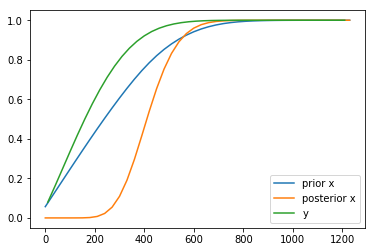计算列车将在 5 分钟内到站的概率：

cdf_y = ete.pmf_y.make_cdf()
print("Probability of train comming in 5 minutes: {:.2f}"
.format(cdf_y.prob(5*60)))

Probability of train comming in 5 minutes: 0.77

# 5. 估计乘客进站速率

如果你刚搬到红线附近，不了解红线地铁的乘客进站速率，可以先假设乘客进站速率在 $[0, 5]$ 之间均匀分布（先验分布）。为了更准确的了解乘客进站速率，你花了 5 天时间，记录每天到车站时的候车人数 $k_1$、等待时间 $y$ 和等待期间进站的乘客数量 $k_2$ 如下：

$k_1$ $y$ $k_2$
17 4.6 9
22 1.0 0
23 1.4 4
18 5.4 12
4 5.8 11

定义表示乘客进站速率的 ArrivalRate 如下：

class ArrivalRate(Suite):
def likelihood(self, data, hypo):
lam = hypo
y, k = data
like = eval_poisson_pmf(lam * y, k)
return like

likelihood() 的参数 data 为等待时间 $y$（y）和等待期间进站的候车人数 $k2$（k），hypo 为乘客进站速率的假设。

定义 ArrivalRateEstimator 对乘客进站速率进行估计：

class ArrivalRateEstimator(object):
def __init__(self, passenger_data):
low, high = 0, 5
n = 51
hypos = np.linspace(low, high, n) / 60

self.prior_lam = ArrivalRate(hypos)
self.post_lam = self.prior_lam.copy()
for k1, y, k2 in passenger_data:
self.post_lam.update((y, k2))

def plot(self):
cdf_prior = self.prior_lam.make_cdf('prior')
cdf_prior.d.index = cdf_prior.d.index * 60
cdf_post = self.post_lam.make_cdf('posterior')
cdf_post.d.index = cdf_post.d.index * 60
cdf_prior.plot_with([cdf_post])

__init__() 中，初始化乘客进站速率的先验分布为在 $[0, 5]$ 上的均匀分布，然后根据 passenger_data 的数据，计算后验概率。注意在 plot() 中对 cdf_priorcdf_post 乘以了 60，是为了将时间单位从人/秒换算到人/分钟。

使用前面的 5 天的数据计算乘客进站速率的后验分布：

data = [[17, 4.6*60, 9], [22, 1.0*60, 0], [23, 1.4*60, 4], [18, 5.4*60, 12], [4, 5.8*60, 11]]

are = ArrivalRateEstimator(data)
print("mean: {:.2f}".format(are.post_lam.mean() * 60))
are.plot()

mean: 2.03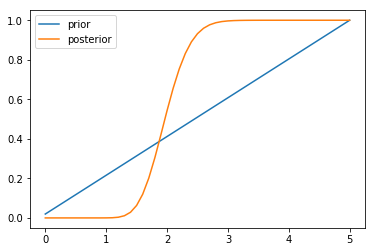# 6. 消除不确定性

在以上的分析中，乘客进站速率 $\lambda$ 是一个不确定的参数，其取值可能会影响到对等待时间 $y$ 的预测。可以按照以下的步骤，评估不确定的参数对分析的影响：

1. 实现基于不确定参数（本例中为 $\lambda$）的确定值分组。
2. 计算不确定参数的分布。
3. 对不确定参数的每个值进行分析，生成一组预测分布。
4. 根据不确定参数的分布，计算预测分布的混合分布。

前面已经完成了 1 和 2 两个步骤，定义 WaitMixtureEstimator 进行步骤 3 和 4：

class WaitMixtureEstimator(object):
def __init__(self, wtc, are, num_passengers=15):
self.meta_pmf = Pmf()
for lam, prob in are.post_lam.iter_items():
if prob <= 0:
continue
ete = ElapsedTimeEstimator(wtc, lam, num_passengers)
self.meta_pmf.set(ete.pmf_y, prob)
self.mixture = make_mixture(self.meta_pmf)

def plot(self, time_limit=600):
plt.figure(figsize=(10, 8))

mix_cdf = self.mixture.make_cdf()
mix_cdf.d = mix_cdf.d.loc[mix_cdf.d.index <= time_limit, ]
plt.plot(mix_cdf.d.index, mix_cdf.d.prob, color='r')

for pmf in self.meta_pmf.values():
cdf = pmf.make_cdf()
cdf.d = cdf.d.loc[cdf.d.index <= time_limit, ]
plt.plot(cdf.d.prob, color='b')

plt.show()

__init__() 的参数 wtc 是前面 WaitTimeCalculator 的对象，are 是前面 ArrivalRateEstimator 的对象。对与 are 中的每个 $\lambda$ 及其概率，通过 ElapsedTimeEstimator 计算等待时间 $y$ 的估计 ete，将 ete 及其对应的 $\lambda$ 的概率保存在 meta_pmf，构造混合概率 mixture

计算过程为：

wme = WaitMixtureEstimator(wtc, are)
wme.plot()

# 7. 决策分析

前面提到，如果发现车站候车的人很多，那么可能列车运行出现了问题，最好打车走。假设为了不迟到，当预计扥待时间超过 15 分钟时，就要选择打车。

使用前面“预测等待时间”一节的分析，可以很容易地得到一个“等待时间超过 15 分钟”关于“车站候车人数”的函数，但这样做的一个问题是，该分析对长时间延误的频次敏感，而长时间延误是一个小概率事件。假设在 1 年期间，你在乘坐红线时遇到了 3 次长时间延误，于是你估计长时间延误的频率是一年 3 次，但这样估计是有偏见的，由于观察者偏差，你更倾向于观察到长时间延误，因为长时间延误影响的人数众多。应该将观察到的长时间延误作为具有偏差的 $zb$ 的样本。

假设你一年乘坐红线 220 次，从列车发车间隔的数据 OBSERVED_GAP_TIMES 中采样 220 个数据，计算 PMF 如下：

n = 220
cdf_z = make_cdf_from_list(OBSERVED_GAP_TIMES)
sample_z = cdf_z.sample(n)
pmf_z = make_pmf_from_list(sample_z)

这里的 pmf_z 是列车实际间隔 $z$，将其偏置为乘客观察到的列车间隔 $zb$，转换为 CDF 的形式，从中采样 n 次，再加上三次时间分别为 30 分钟（1800 秒）、40 分钟（2400 秒）和 50 分钟（3000 秒）的长时间延误：

cdf_zb = bias_pmf(pmf_z).make_cdf()
sample_zb = cdf_zb.sample(n) + [1800, 2400, 3000]

然后，使用加入了长时间延误数据的 sample_zb 估计 $zb$ 的 PDF，并转换为 PMF：

pdf_zb = EstimatedPdf(sample_zb)
xs = make_range(low=60)
pmf_zb = pdf_zb.make_pmf(xs)

最后，对 pmf_zb 进行反偏置，得到引入了长时间延误的 $z$ 的 PMF，使用 WaitTimeCalculator 计算等待时间：

pmf_z = unbias_pmf(pmf_zb)
wtc = WaitTimeCalculator(pmf_z)

def unbias_pmf(pmf, name=''):
pmf_new = pmf.copy(name=name)
for x, p in pmf.iter_items():
pmf_new.mult(x, 1.0 / x)
pmf_new.normalize()
return pmf_new

使用如下代码计算不同候车人数的情况下，等待时间超过 15 分钟的概率：

lst_num_passengers = [0, 5, 10, 15, 20, 25, 30, 35]
lst_probs = []
for num_passengers in lst_num_passengers:
ete = ElapsedTimeEstimator(wtc, num_passengers=num_passengers)
cdf_y = ete.pmf_y.make_cdf()
prob = 1 - cdf_y.prob(15 * 60)
lst_probs.append(prob)

plt.figure()
plt.plot(lst_num_passengers, lst_probs)
plt.plot()